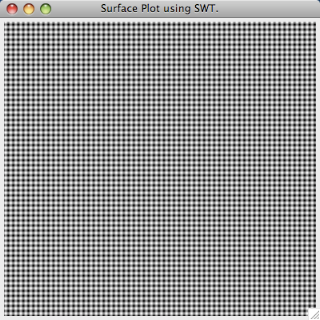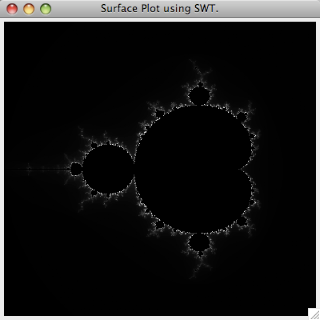Wednesday, September 24, 2008

Plotting 2-D Surfaces Using Java SWTIf you are creating an RCP application in Eclipse or just using Java's SWT graphics packages for your program, there may be a need to make a surface plot to display some data contained in a matrix. Rather than learning an additional graphics package like Java 2D or JFreeChart, if you're already using SWT as the basis of your application, it's best just stick with that. It's also incredibly easy to do as demonstrated in the code snippet below. The main method creates a Shell which contains the 2-D surface plot. The createContents method creates a Canvas object, and within the PaintListener, it colors each pixel according the matrix returned by getMatrix. The constructor for the Color object takes and RGB value, and you need to be sure that your matrix only includes integers between 0 and 255 or it won't work. The getMatrix method just returns a 2-D matrix of integers. Just for demonstration purposes, I came up with three simple example matrices. The matrix plotted to the left is just random noise scaled between 0 and 255 using matrix[i][j] = (int)(Math.random()*255) in the getMatrix method.

Here's the entire snippet:

``package com.timmolter.samples;import org.eclipse.swt.SWT;import org.eclipse.swt.events.PaintEvent;import org.eclipse.swt.events.PaintListener;import org.eclipse.swt.graphics.Color;import org.eclipse.swt.layout.GridData;import org.eclipse.swt.layout.GridLayout;import org.eclipse.swt.widgets.Canvas;import org.eclipse.swt.widgets.Display;import org.eclipse.swt.widgets.Shell;public class SurfacePlotter { static int size = 400; public static void main(String[] args) {      Display display = new Display();      Shell shell = new Shell(display);      shell.setText("Surface Plot using SWT.");      createContents(shell);      shell.setSize(size, size);      shell.open();      while( !shell.isDisposed())         {           if(!display.readAndDispatch())           display.sleep();         }      display.dispose(); } private static void createContents(final Shell shell) {       // GridLayout for shell     GridLayout gridLayoutMain = new GridLayout();     gridLayoutMain.makeColumnsEqualWidth = true;     shell.setLayout(gridLayoutMain);       // Surface Canvas     final Canvas surfaceCanvas = new Canvas(shell, SWT.NONE);     surfaceCanvas.addPaintListener(new PaintListener(){         public void paintControl(PaintEvent e) {                       int[][] matrix = getMatrix(surfaceCanvas.getSize().x, surfaceCanvas.getSize().y);                       for(int i=0; i < matrix.length; i++){                 for(int j=0; j < matrix.length; j++){                     e.gc.setForeground(new Color(e.display, matrix[i][j], matrix[i][j], matrix[i][j])); //rgb                      e.gc.drawPoint(i, j);                 }             }         }     });       // GridData for surfaceCanvas     GridData data = new GridData(GridData.FILL_BOTH);     surfaceCanvas.setLayoutData(data);       } private static int[][] getMatrix(int xSize, int ySize){       int[][] matrix = new int[xSize][ySize];     for (int i = 0; i < xSize; i++) {         for (int j = 0; j < ySize; j++) {             matrix[i][j] = (int)(Math.random()*255);             //matrix[i][j] = (int)((.5*(Math.sin(j)+1))*255);             //matrix[i][j] = (int)(((.25*(Math.sin(i)+1))+(.25*(Math.cos(j)+1)))*255);         }     }     return matrix; }}``matrix[i][j] = (int)(((.25*(Math.sin(i)+1))+(.25*(Math.cos(j)+1)))*255);

The Mandelbrot Set, which I previously blogged about here, is a more interesting surface to look at. To plot the Mandelbrot Set, you just need to replace the getMatrix method in createContents with getMandelbrotSet. The getMandelbrotSet method is as follows:

``private static int[][] getMandelbrotSet(int xSize, int ySize) { int[][] mandelbrotSet = new int[xSize][ySize]; int n = 0; int nmax = 255; double x,y,xnew,ynew,z2,cx,cy; for(int i = 0; i<xSize; i++){     for(int j = 0; j<ySize; j++){         cx=-2.0+(3.*i)/xSize;         cy=1.5-(3.*j)/ySize;         n = 0;         x = 0;         y = 0;         z2 = 0;         while(n < nmax && z2 < 4){             xnew = x*x-y*y+cx;             ynew = 2*x*y+cy;             z2 = xnew*xnew+ynew*ynew;             x = xnew;             y = ynew;             n++;         }         if (n == nmax){             mandelbrotSet[i][j] = 0;         }         else{             mandelbrotSet[i][j] = n;         }     } } return mandelbrotSet;}``

The resulting 2-D surface looks like this:BTW, many thanks to Greg Houston for providing the JavaScript web application I used to convert my source code into HTML format for inserting into my blog.

avanrotciv said...

Hey man, can you tell me wher can I find:

com.timmolter.samples

I'm also getting an error here:

final Canvas surfaceCanvas = new Canvas(shell, SWT.NONE);

error: Can't resolve for SWT

I like your copyright policy! after you share something on the internet how can you control what people do with it?Anonymous said...

Thank you very much! It's work realy good!

Tim Molter said...